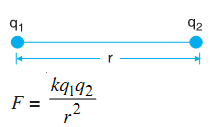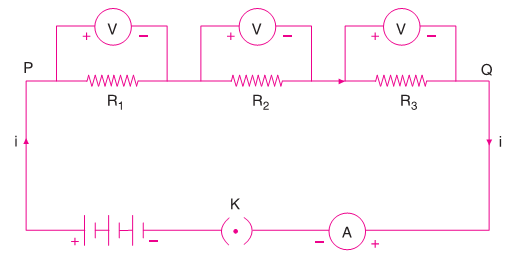# Electricity

A plastic comb when brought near a piece of paper does not pick up small pieces of a paper. But if you comb your dry hair and bring the comb close to a small piece of paper, you will notice that the bits of paper are attracted towards the comb. This happens because the comb gets charged or electrified when you comb your dry hair.

### Electrostatic Force

According to Coulomb’s law, the magnitude of the force of attraction (or repulsion) between two point charges is directly proportional to the product of the quantity of two charges and inversely proportional to the square of the distance between them.k is a constant of proportionality depending upon the nature of the medium in which the charges are placed.

k = 9 × 109 N m2 C-2 for vacuum (or air).

1 C is the charge when placed at a distance of 1 m from an equal like charge in vacuum, experiences a repulsive force of 1 N. Force is directed along the line joining the centres of the two charges. For like charges force is repulsive (positive in sign), while for unlike charges it is attractive (with negative sign).

### Electrostatic Potential

Consider an uncharged body like a glass rod which is given a certain charge (say a positive charge), the body acquires that charge. Now if you add more charge of the same nature on it, the charge will experience a force of repulsion due to already existing charge on it.

Therefore, some work has to be done by any external  agent to overcome this force of repulsion. This work is stored up as electrostatic potential energy in the system of charges. This is analogous to the process of raising a body above the ground against the force of attraction in which work done against gravity is stored in the body as its gravitational potential energy.

Let a charge q be moved upto a distance r towards a source charge Q, the electrostatic potential energy possessed by charge q is given by

U = kQq/r

The electrostatic potential (or potential) at any point in the vicinity of a charge is defined as the amount of work done in bringing a unit positive charge from infinity to that point. If W is the work done in bringing a positive charge q from infinity to a point in the vicinity of source charge Q, the potential V at the point due to charge Q is

V = W/q

U/q = kQ/r

Electrostatic potential is a scalar quantity (It has only magnitude and no direction). Its SI unit is joule/coulomb (JC-1) or volt (V).

The potential at a point is 1 V if +1 C charge placed at that point possesses a potential energy of 1 J or the potential at a point is 1 V if 1 J of work is done in bringing 1 C of positive charge from infinity to that point.

1 volt = 1 joule / 1 coulomb

### Potential Difference

Consider a charge q is placed at a point. Let B and C be two points where point B is closer to q than C. If a charge q is brought from infinity to C or from infinity to B work done respectively be WC and WB. The potential at points B and C respectively:

VB = WB/q

VC = WC/q

The potential difference is the difference in potentials VB and VC:

VB - VC = (WB - WC)/q

WB - WC is the work done in carrying charge from point C to B.

Thus potential difference between two points B and C is equal to the amount of work done in moving a unit charge from point C to point B.

V = W/q

The potential difference (pd) between two points of a conductor is said to be 1 volt if 1 joule of work is done in moving 1 coulomb of charge from one point to another. Potential difference is a scalar quantity. It is measured using an instrument voltmeter. Voltmeter is always connected in parallel across which you have to measure the potential difference.

### Electric Current

Electric current is the charge flowing through any cross section of the conductor in a unit time.

i = charge (Q) / time (t)

Q is the charge in coulomb flowing through the conductor in t seconds. If 1 coulomb (C) of charge flows through any cross section of a conductor in 1 second (s), the current flowing it will be 1 ampere (A).

An ammeter is an instrument which on connecting in series in an electrical circuit indicates how many amperes of current is flowing in the electric circuit.

### Electric Cell

A cell is a device in which chemical energy is converted into electrical energy. In the cell negatively charged plate repels the electrons which causes the electrons to move along the wire. Hence the electrons flow from the negatively charged plate through the wire to positively charged plate of the cell.

Conventionally the direction of the current is taken as opposite to the direction of the flow of electrons i.e., from the positive to the negative terminal.The combination of cells is called a battery.

### Conductors and Insulators

All materials can be divided into two categories on the basis of movement of charges through them: conductors and insulators.

Conductors are the materials which allow the electric current to flow through them quite freely. For example, metals like silver, copper, aluminum.

Insulators are the materials which do not allow electricity to flow through them freely. for example, rubber, glass, bakelite.

### Resistors

The electrical resistance is the tendency to resist the flow of electric current. A wire having a desired resistance for use in an electric circuit is called a resistor.

Resistance can be both either desirable or undesirable in a conductor or circuit. In a conductor, to transmit electricity from one place to another place, the resistance is undesirable. Resistance in a conductor causes part of electrical energy to turn into heat, so some electrical energy is lost along the path.

On the other hand it is the resistance which allows to use electricity for light and heat.

### Ohm's Law

The current flowing through a wire is directly proportional to the potential difference applied across its ends.

V ∝ i

V = Ri

Ohm’s Law states that the current flowing through a conductor is directly proportional to the potential difference applied across the ends of the conductor provided temperature of the conductor remains the same.

### Resistance of Wire

Resistance of wire is a constant for a given wire. It is directly proportional to the length and inversely proportional to the cross-sectional area.

Resistance is a scalar quantity and its SI unit is ohm denoted by the symbol Ω (omega). 1 ohm is the resistance of a wire across which when 1 V potential difference is applied, 1 A current flows through the wire.

1 ohm = 1 volt / 1 ampere

### Combination of Resistors

In an electric circuit, resistors can be connected in two different ways:

1. Series Combination: two or more resistors are combined end to end consecutively.
2. Parallel Combination: two or more resistors can be connected between the same two points.

Series Combination

When resistors are connected in series with a cell and an ammeter, due to one path the same current i flows through all of them.Let the potential difference between the ends of the resistors R1, R2 and R3 are respectively V1, V2 and V3. By ohm’s law potential difference across each resistor

V1 = iR1

V2 = iR2

V3 = iR3

If the potential difference between P and Q be V, then

V = V1 + V2 + V3

V = iR1 + iR2 + iR3

V = i(R1 + R2 + R3)

Let total or equivalent resistance between P and Q is Rs

V = iRs

Rs = R1 + R2 + R3

The equivalent resistance of three resistors connected in series is equal to the sum of their individual resistances.

Parallel Combination

When three resistors connected in parallel with a cell and an ammeter, the potential difference between points P and Q is same across each resistor but the current flows from P to Q is equal to the sum of the separate currents passing through each branch of a given resistance.If i1, i2 and i3 respectively represent the current passing through the branches having the resistors R1, R2, and R3 then the total current i in the main circuit is

i = i1 + i2 + i3

If V is the potential difference across each of the resistors, then according to Ohm’s law

i1 = V/R1

i2 = V/R2

i3 = V/R3

If RP is the equivalent resistance of the resistors connected in parallel having the same potential difference V then

i = V/Rp

V/Rp = V/R1 + V/R2 + V/R3

1/Rp = 1/R1 + 1/R2 + 1/R3

The sum of the reciprocals of the separate resistances is equal to the reciprocal of equivalent or total or resultant resistor Rp.

### Heating Effect of Electric Current

Consider a conductor XY of resistance R. Let current ‘i’ is passed for t seconds through the conductor on applying a potential difference V across the ends X and Y. If the charge Q is to be transferred from point X to Y, the work is done in moving the charge Q across the ends of the conductor. Work done in transferring the charge Q,

W = potential difference (V) × Charge (Q)

Q = it

W = Qit

According to Ohm’s law, V = iR

W = (iR)it

W = i2Rt

The work done in moving the electric charge across a resistance appears in the form of heat. Therefore, the heat produced in the conductor is

H = i2Rt

The amount of heat produced in a conductor on passing the current i is directly proportional to the square of the current (i2), the resistance of the conductor (R) and the time (t) for which the current flows through the conductor. This is known as Joule’s law of heating.

### Electric Power

The rate at which electric energy is consumed or dissipated is termed as electric power.

Electric power (P) = Work done (W) / Time taken (t)

P = Vit/t

P = Vi

P = (iR)i

P = i2R

P = (V/R)2R

P = V2/R

SI unit of electric power is joule/second or watt (W).

Electric power consumed in a circuit or a device is 1 W if a current of 1 A flows through it when a potential difference of one volt is maintained across it.